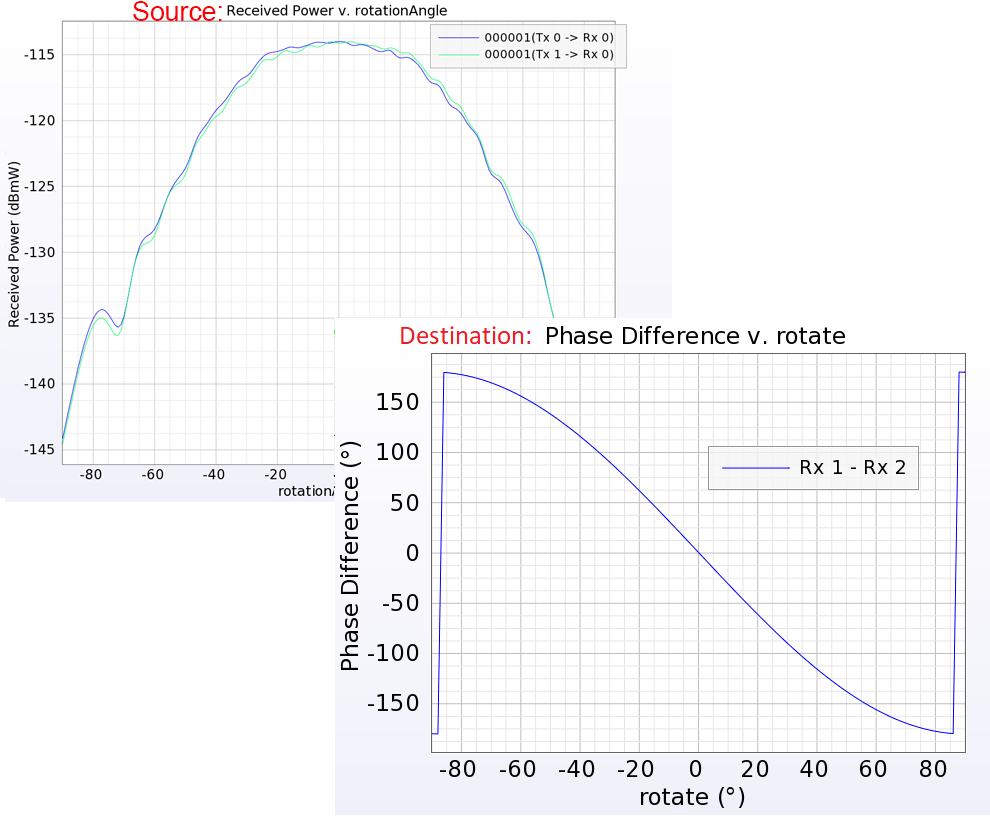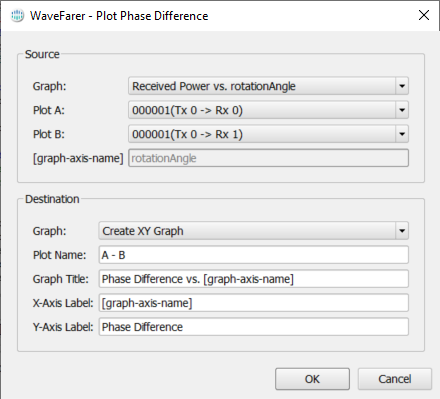# Plot Phase Difference | WaveFarer

Compute the phase difference between two plots.

The Plot Phase Difference script allows users to select two plots from an existing graph and compute the angular difference between them. It is a fully featured script with an interactive user interface.

## Use CaseInconsistencies may exist between the measurement setup and user-defined antenna in WaveFarer. This script can be used as a part of one import verification method, which compares the phase difference between the two receivers of the physical prototype to that of the WaveFarer simulation. Users can reference the User-Defined Antennas tutorial for an example.

The script can be applied to any graph that meets the following conditions:

• It exists in the project tree.
• It is an XY graph.
• It contains two or more plots.
• It has an a-axis with length or angle units.
• It has a y-axis with an angle unit.

## Workflow

Execute the macro using the following steps: